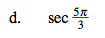### Home > CALC > Chapter 1 > Lesson 1.4.2 > Problem1-158

1-158.
1. Without using a calculator, find the values of each of the following trig expressions. Homework Help ✎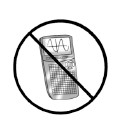1.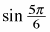2.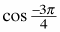3.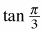4.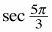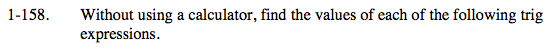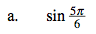Visualize the angle on the unit circle. What side ratio correspond with that angle? What quadrant is it in? (This will determine whether your answer will be positive or negative.)

$\frac{1}{2}$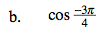See hint in part (a).

$\text{Recall that tan}(a)=\frac{\text{sin}(a)}{\text{cos}(a)}$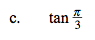$\text{Recall that sec}(a)=\frac{1}{\text{cos}(a)}$